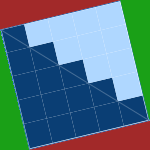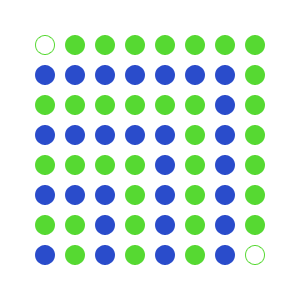Hey everyone, I'm starting a new article series here on catonmat that will be published on Fridays. It's called Visual Math Friday. The goal of this article series is to assemble all the wonderful mathematical proofs and identities that can be proven in a single picture or drawing. I have been collecting them for years from various books, math journals, websites and video lectures and have collected several hundred of them.

If you have been following my blog, you'll have noticed that I love to explain things. I'll use the same writing style in this series as well. I'll post the visual proof together with my explanation. In the explanations I'll walk you through the proof, suggesting how to think about the visual image. But it's best if you try to understand the proof without reading my explanation and only then read it.

The proofs come from algebra, number theory, geometry, calculus, complex analysis, topology and various other fields of mathematics. I'll do one proof a week and will try to alternate between really easy, moderate and difficult proofs every week so that readers with various mathematical backgrounds can enjoy the series.

I'll start with a really easy integer sum proof this week. Without further ado, here it is.Now try to figure what it proves without reading further! The colors play an important role in the visual proofs, as do the shape of the visual drawing, the shapes on it and their arrangement!

## The Proof

This picture proves that the sum of first n odd numbers is equal to n². In other words:

1 + 3 + 5 + 7 + 9 + 11 + ... + (2n-1) = n².

## Explanation

Imagine that the blue and green dots are marbles. Now let's look at the lower left corner. One blue marble is located there. Next if we follow the diagonal to the upper right corner, we see that the 1 blue marble is kind-of wrapped in 3 green marbles, then they in turn are wrapped in 5 blue marbles again, then those are wrapped in 7 green ones, which in turn are wrapped in 9 blue ones, then 11 green ones, and finally 13 ones.

This suggests that we could look at the sum 1 + 3 + 5 + 7 + 9 + 11 + 13. Well what is it? It's 49. Also what does this sum involve? It involves only odd numbers. Interesting.

Now let's count how many marbles we have on each side. 7 on one side and 7 on the other. And what is 7x7? It's 49! And how many numbers did we sum together? Well, seven - 1, 3, 5, 7, 9, 11, 13. Interesting.

Could it be that the sum of the first n odd numbers is equal to n²? Or in other words, is 1 + 3 + 5 + 7 + ... + (2n-1) equal to n²?

Let's try to prove this hypothesis by going one number further and adding 15 green marbles - 13 filled marbles and 2 semi-filled marbles:Adding 15 marbles created a square of marbles again - now we have 64 marbles, 8 on each side.

The 2 semi-filled marbles are very important here. Each time we go further from n odd marbles to n+1 odd marbles, the difference changes by 2. This difference of two marbles can always be put where the semi-filled ones are in this picture.

This actually concludes the proof. We showed that the proof worked for several marbles, and showed how it still works if we went one step further. Therefore it works for all future steps. This method of proof is called mathematical induction and it's a very powerful and important tool in mathematics for proving sums like these.

## Before we go

Can you figure out the two proofs hidden in the logo of this post?Let me know in the comments if you can!